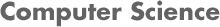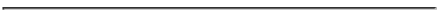### CS 548 KNOWLEDGE DISCOVERY AND DATA MINING - Fall 2018 Project 2: Decision Trees, Linear Regression, Model Trees, Regression Trees

#### PROF. CAROLINA RUIZ

DUE DATE: Thursday October 11th, 2018.
• Slides: Submit via Canvas by 2:00 pm.
• Written report: Hand in a hardcopy by the beginning of class (by 3:59 pm).### Project Assignment:

1. Study Chapter 3, Sections 10.1, 10.3 and Appendix D (online) of the textbook in great detail.

2. Study decision tree prunning using the Weka book: Section 6.1.

3. Study linear regression, model trees and regression trees using the Weka book: Sections 3.2, 3.3, 4.6 and
• If you have Witten's, Frank's, and Hall's 3rd edition of the book (available on reserve in the WPI Library under "CS548"): Section 6.6 (Numeric Predictions with Local Linear Models).
• If you have Witten's, Frank's, Hall's and Pal's 4th edition of the book: Section 7.3 (Numeric Predictions with Local Linear Models).

4. Study all the materials posted on the course Lecture Notes:
In particular, you should know the algorithms to construct decision trees, regression trees, and model trees very well, and be able to use these algorithms to construct trees from data by hand during the test. See examples provided in the Lecture Notes linked above. (Note: for model and regression trees, a software tool will be used to obtain the necessary linear regressions.)

5. THOROUGHLY READ AND FOLLOW THE PROJECT GUIDELINES. These guidelines contain detailed information about how to structure your project, and how to prepare your written summary, and how to study for the test.

*** You must use the Project 2 Template provided here for your written report. Do NOT change the structucture of the report, do NOT exceed the page limits stated in the template and do NOT decrease the font size ***. (If you prefer not to use Word, you can copy and paste this format in a different editor as long as you respect the stated page structure and page limit.)

• Data Mining Technique(s): Run experiments in Weka AND in Python using the following techniques:

• Pre-processing Techniques: Feature selection, feature creation, dimensionality reduction, noise reduction, attribute discretization, ...

• Classification Techniques:
• Zero-R (majority class)
• One-R
• Decision trees: Using Weka (J4.8) and Python.
Since these decision tree implementations are able to handle continuous attributes and missing values directly, make sure to run some experiments with no pre-processing and some experiments with pre-processing (discretizing continuous attributes and replacing missing values before hand), and compare the results.

• Regression Techniques:
• Linear Regression: Weka (under "functions") and Python.
• Regression Trees: Weka (M5P under "trees") and Python.
• Model Trees: Weka (M5P under "trees") and Python.

• Dataset: Use the Absenteeism at work Data Set. This dataset is available at the UCI Machine Learning Repository.

• Use "Absenteeism_time_in_hours" as the target attribute.

• Before you run experiments, transform the dataset as follows:
1. Change the name of the "Son" attribute to "Children".
2. For classification experiments:
• Discretize the "Absenteeism_time_in_hours" into 6 equal-frequency bins (you can use Weka for this using the unsupervised discretize filter and then save the filtered dataset). The bins should be the following:
```(-inf-1.5]
(1.5-2.5]
(2.5-3.5]
(3.5-7.5]
(7.5-12]
(12-inf)
```
3. For regression experiments:
• Use the "Absenteeism_time_in_hours" target attribute as originally provided in the dataset (i.e., continuous).

• Run experiments with and without discretizing the predicting attributes (i.e., independent variables); removing attributes that are too related to the target or that make the trees too long; and with any other pre-processing and post-processing that produce useful and meaningful models.

• Performance Metric(s):
• Use the following metrics or evaluation methods:
1. For classification tasks: use classification accuracy, precision, recall, ROC Area, and confusion matrices.
For regression tasks: use correlation coefficient AND any subset of the following error metrics that you find appropriate: mean-squared error, root mean-squared error, mean absolute error, relative squared error, root relative squared error, and relative absolute error. An important part of the data mining evaluation in this project is to try to make sense of these performance metrics and to become familiar with them.
2. size of the tree,
3. readability of the tree, as a separate qualitative criterion to evaluate the "goodness" of your models, and
4. time it took to construct the tree.
• Compare each accuracy/error you obtained against those of benchmarking techniques as ZeroR and OneR over the same (sub-)set of data instances you used in the corresponding experiment.
• Remember to experiment with pruning of your tree: Experiment with pre- and/or post-prunning of the tree in order to increase the classification accuracy, reduce the prediction error, and/or reduce the size of the tree.

• Advanced Topic(s): Investigate more depth (experimentally, theoretically, or both) a topic of your choice that is related to decision or model/regression trees and that was not covered already in this project, class lectures, or the textbook. This tree-related topic might be something that was described or mentioned briefly in the textbook or in class; comes from your own research; or is related to your interests. Just a few sample ideas are: The prune functions in Python; C4.5; C4.5 pruning methods (for trees or for rules); any of the additional tree classifiers in Weka: DecisionStump, LMT RandomForest, RandomTree, REPTree; meta-learning applied to decision trees (see Classifier -> Choose -> meta); other useful functionality in Python; an idea from a research paper that you find intriguing; or any other tree-related topic.